Courses

Integer Answer Type Questions: Motion | JEE Advanced Notes | Study Physics 35 Years JEE Main & Advanced Past year Papers - JEE

JEE: Integer Answer Type Questions: Motion | JEE Advanced Notes | Study Physics 35 Years JEE Main & Advanced Past year Papers - JEE

The document Integer Answer Type Questions: Motion | JEE Advanced Notes | Study Physics 35 Years JEE Main & Advanced Past year Papers - JEE is a part of the JEE Course Physics 35 Years JEE Main & Advanced Past year Papers.
All you need of JEE at this link: JEE

Q.1. A train is moving along a straight line with a constant acceleration ‘a’. A boy standing in the train throws a ball forward with a speed of 10 m/s, at an angle of 60° to the horizontal. The boy has to move forward by 1.15 m inside the train to catch the ball back at the initial height. The acceleration of the train, in m/s2, is

Ans. 5

Solution. From t h e perspective of observer A, consider in g vertical motion of the ball from the point of throw till it reaches back at the initial height.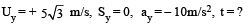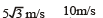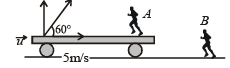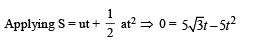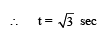Considering horizontal motion from the perspective of observer B. Let u be the speed of train at the time of throw.

The horizontal distance travelled by the ball = (u + 5) √3 .

The horizonal distance travelled by the boy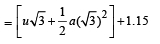As the boy catches the ball therefore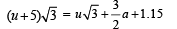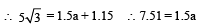∴   a ≈5 m/s2

Q.2. Airplanes A and B are flying with constant velocity in the same vertical plane at angles 30° and 60° with respect to the horizontal respectively as shown in figure. The speed of A is 100 √3 m/s. At time t = 0 s, an observer in A finds B at a distance of 500 m. The observer sees B moving with a constant velocity perpendicular to the line of motion of A. If at t = t0, A just escapes being hit by B, t0 in seconds is         (JEE Adv. 2014)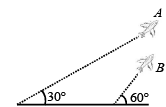Ans. 5

Solution.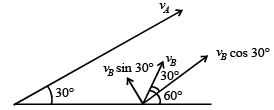Here

vA = vB cos 30°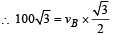∴vB = 200 ms–1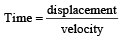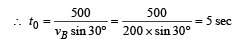Q.3. A rocket is moving in a gravity free space with a constant acceleration of 2 m/s2 along +x direction (see figure). The length of a chamber inside the rocket is 4 m. A ball is thrown from the left end of the chamber in +x direction with a speed of 0.3 m/s relative to the rocket. At the same time, another ball is thrown in –x direction with a speed of 0.2 m/s from its right end relative to the rocket. The time in seconds when the two balls hit each other is      (JEE Adv. 2014)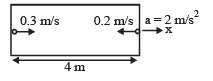Ans. 8

Solution.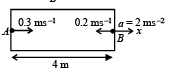For ball A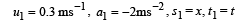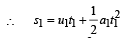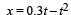...(1)

For ball B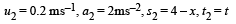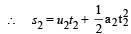4 – x = 0.2 t + t2 ...(2)

From (1) and (2) t = 8 sec

The document Integer Answer Type Questions: Motion | JEE Advanced Notes | Study Physics 35 Years JEE Main & Advanced Past year Papers - JEE is a part of the JEE Course Physics 35 Years JEE Main & Advanced Past year Papers.
All you need of JEE at this link: JEEUse Code STAYHOME200 and get INR 200 additional OFF Use Coupon Code

Top Courses for JEEPhysics 35 Years JEE Main & Advanced Past year Papers

88 docs|49 tests

Top Courses for JEETrack your progress, build streaks, highlight & save important lessons and more!

,

,

,

,

,

,

,

,

,

,

,

,

,

,

,

,

,

,

,

,

,

;